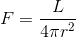# Luminosity Equation Question

Hello,

I've been trying to solve for the luminosity of Gliese 581 using its brightness. I've been using the equation below:F = (L/(4*pi*r^2))

I have the flux as the absolute brightness of Gliese 581, which is 11.6. The distance, r, to the star is 1.89210568 × 10^17 meters. However, when I input all of that into the equation, I get 5.21 * 10^36 watts. That does not seem even close to the correct answer, which should be about 6.38 * 10^24 watts. What did I do wrong?

F = (L/(4*pi*r^2)) I have the flux as the absolute brightness of Gliese 581, which is 11.6.
In which units?
The distance, r, to the star is 1.89210568 × 10^17 meters. However, when I input all of that into the equation, I get 5.21 * 10^36 watts. That does not seem even close to the correct answer, which should be about 6.38 * 10^24 watts. What did I do wrong?
You need F in units if W/mˇ2
How do you get that?
Because that "absolute brightness 11,6" is in different units - not W/m2.

How can I convert the absolute brightness of 11.6 into flux?